概述

TreeMap实现了SotredMap接口，它是有序的集合。而且是一个红黑树结构，每个key-value都作为一个红黑树的节点。如果在调用TreeMap的构造函数时没有指定比较器，则根据key执行自然排序。这点会在接下来的代码中做说明，如果指定了比较器则按照比较器来进行排序。

数据结构

继承关系

public class TreeMap<K,V>
extends AbstractMap<K,V>
implements NavigableMap<K,V>, Cloneable, java.io.Serializable {}

实现接口

Serializable, Cloneable, Map<K,V>, NavigableMap<K,V>, SortedMap<K,V>

基本属性

private final Comparator<? super K> comparator;  //比较器，是自然排序，还是定制排序 ，使用final修饰，表明一旦赋值便不允许改变
private transient Entry<K,V> root = null;  //红黑树的根节点
private transient int size = 0;     //TreeMap中存放的键值对的数量
private transient int modCount = 0;   //修改的次数

源码解析

数据结构

static final class Entry<K,V> implements Map.Entry<K,V> {
K key;    //键
V value;    //值
Entry<K,V> left = null;     //左孩子节点
Entry<K,V> right = null;    //右孩子节点
Entry<K,V> parent;          //父节点
boolean color = BLACK;      //节点的颜色，在红黑树种，只有两种颜色，红色和黑色

//构造方法，用指定的key,value ,parent初始化，color默认为黑色
Entry(K key, V value, Entry<K,V> parent) {
this.key = key;
this.value = value;
this.parent = parent;
}

//返回key
public K getKey() {
return key;
}

//返回该节点对应的value
public V getValue() {
return value;
}

//替换节点的值，并返回旧值
public V setValue(V value) {
V oldValue = this.value;
this.value = value;
return oldValue;
}
//重写equals()方法
public boolean equals(Object o) {
if (!(o instanceof Map.Entry))
return false;
Map.Entry<?,?> e = (Map.Entry<?,?>)o;
//两个节点的key相等，value相等，这两个节点才相等
return valEquals(key,e.getKey()) && valEquals(value,e.getValue());
}
//重写hashCode()方法
public int hashCode() {
int keyHash = (key==null ? 0 : key.hashCode());
int valueHash = (value==null ? 0 : value.hashCode());
//key和vale hash值得异或运算，相同则为零，不同则为1
return keyHash ^ valueHash;
}
//重写toString()方法
public String toString() {
return key + "=" + value;
}
}

构造方法

//构造方法，comparator用键的顺序做比较
public TreeMap() {
comparator = null;
}

//构造方法，提供比较器，用指定比较器排序
public TreeMap(Comparator<? super K> comparator) {
his.comparator = comparator;
}

//将m中的元素转化daoTreeMap中，按照键的顺序做比较排序
public TreeMap(Map<? extends K, ? extends V> m) {
comparator = null;
putAll(m);
}

//构造方法，指定的参数为SortedMap
//采用m的比较器排序
public TreeMap(SortedMap<K, ? extends V> m) {
comparator = m.comparator();
try {
buildFromSorted(m.size(), m.entrySet().iterator(), null, null);
} catch (java.io.IOException cannotHappen) {
} catch (ClassNotFoundException cannotHappen) {
}
}

TreeMap提供了四个构造方法，实现了方法的重载。无参构造方法中比较器的值为null,采用自然排序的方法，如果指定了比较器则称之为定制排序.

• 自然排序：TreeMap的所有key必须实现Comparable接口，所有的key都是同一个类的对象
• 定制排序：创建TreeMap对象传入了一个Comparator对象，该对象负责对TreeMap中所有的key进行排序，采用定制排序不要求Map的key实现Comparable接口。等下面分析到比较方法的时候在分析这两种比较有何不同。

put()

public V put(K key, V value) {
Entry<K,V> t = root;     //红黑树的根节点
if (t == null) {        //红黑树是否为空
compare(key, key); // type (and possibly null) check
//构造根节点，因为根节点没有父节点，传入null值。
root = new Entry<>(key, value, null);
size = 1;     //size值加1
modCount++;    //改变修改的次数
return null;    //返回null
}
int cmp;
Entry<K,V> parent;    //定义节点
// split comparator and comparable paths
Comparator<? super K> cpr = comparator;     //获取比较器
if (cpr != null) {      //如果定义了比较器，采用自定义比较器进行比较
do {
parent = t;      //将红黑树根节点赋值给parent
cmp = cpr.compare(key, t.key);     //比较key, 与根节点的大小
if (cmp < 0)      //如果key < t.key , 指向左子树
t = t.left;   //t = t.left  , t == 它的做孩子节点
else if (cmp > 0)
t = t.right;  //如果key > t.key , 指向它的右孩子节点
else
return t.setValue(value);      //如果它们相等，替换key的值
} while (t != null);        //循环遍历
}
else {
//自然排序方式，没有指定比较器
if (key == null)
throw new NullPointerException();  //抛出异常
Comparable<? super K> k = (Comparable<? super K>) key;    //类型转换
do {
parent = t;
cmp = k.compareTo(t.key);
if (cmp < 0)     // key < t.key
t = t.left;   //左孩子
else if (cmp > 0)   // key > t.key
t = t.right;    //右孩子
else
return t.setValue(value);   //t == t.key , 替换value值
} while (t != null);
}
Entry<K,V> e = new Entry<>(key, value, parent);   //创建新节点，并制定父节点
//根据比较结果，决定新节点为父节点的左孩子或者右孩子
if (cmp < 0)
parent.left = e;
else
parent.right = e;
fixAfterInsertion(e);   //新插入节点后重新调整红黑树
size++;
modCount++;
return null;
}
//比较方法，如果comparator==null ,采用comparable.compartTo进行比较，否则采用指定比较器比较大小
final int compare(Object k1, Object k2) {
return comparator==null ? ((Comparable<? super K>)k1).compareTo((K)k2)
: comparator.compare((K)k1, (K)k2);
}

private void fixAfterInsertion(Entry<K,V> x) {
//插入的节点默认的颜色为红色
x.color = RED;    //
//情形1： 新节点x 是树的根节点，没有父节点不需要任何操作
//情形2： 新节点x 的父节点颜色是黑色的，也不需要任何操作

while (x != null && x != root && x.parent.color == RED) {
//情形3：新节点x的父节点颜色是红色的
//判断x的节点的父节点位置，是否属于左孩子
if (parentOf(x) == leftOf(parentOf(parentOf(x)))) {
//获取x节点的父节点的兄弟节点，上面语句已经判断出x节点的父节点为左孩子，所以直接取右孩子
Entry<K,V> y = rightOf(parentOf(parentOf(x)));
//判断是否x节点的父节点的兄弟节点为红色。
if (colorOf(y) == RED) {
setColor(parentOf(x), BLACK); // x节点的父节点设置为黑色
setColor(y, BLACK);           // y节点的颜色设置为黑色
setColor(parentOf(parentOf(x)), RED); // x.parent.parent设置为红色
x = parentOf(parentOf(x)); // x == x.parent.parent ,进行遍历。
} else {
//x的父节点的兄弟节点是黑色或者缺少的
if (x == rightOf(parentOf(x))) {   //判断x节点是否为父节点的右孩子
x = parentOf(x);     //x == 父节点
rotateLeft(x);    //左旋转操作
}
//x节点是其父的左孩子
setColor(parentOf(x), BLACK);
setColor(parentOf(parentOf(x)), RED);  //上面两句将x.parent 和x.parent.parent的颜色做调换
rotateRight(parentOf(parentOf(x)));   //进行右旋转
}
} else {
Entry<K,V> y = leftOf(parentOf(parentOf(x)));  //y 是x 节点的祖父节点的左孩子
if (colorOf(y) == RED) {    //判断颜色
setColor(parentOf(x), BLACK);    //父节点设置为黑色
setColor(y, BLACK);         //父节点的兄弟节点设置为黑色
setColor(parentOf(parentOf(x)), RED);   //祖父节点设置为红色
x = parentOf(parentOf(x));   //将祖父节点作为新插入的节点，遍历调整
} else {
if (x == leftOf(parentOf(x))) {     //x 是其父亲的左孩子
x = parentOf(x);
rotateRight(x);    //以父节点为旋转点，进行右旋操作
}
setColor(parentOf(x), BLACK);    //父节点为设置为黑色
setColor(parentOf(parentOf(x)), RED);  //祖父节点设置为红色
rotateLeft(parentOf(parentOf(x)));  //以父节点为旋转点，进行左旋操作
}
}
}
root.color = BLACK; //通过节点位置的调整，最终将红色的节点条调换到了根节点的位置，根节点重新设置为黑色
}

1. 每个节点只能是红色或者黑色
2. 根节点永远是黑色的
3. 所有的叶子的子节点都是空节点，并且都是黑色的
4. 每个红色节点的两个子节点都是黑色的（不会有两个连续的红色节点）
5. 从任一个节点到其子树中每个叶子节点的路径都包含相同数量的黑色节点（叶子节点到根节点的黑色节点数量每条路径都相同）

• 情形1：新插入的节点时红黑树的根节点，没有父节点，无需任何的操作，直接将颜色设置为黑色就可以了

• 情形2：新节点的父节点颜色是黑色的，新插入的节点是红色的。也无需任何的操作。因为新节点的插入并没有影响到红黑书的特点

• 情形3：新节点的父节点（左孩子节点）颜色是红色的，而父节点的兄弟节点颜色也是红色的。那么情况就出现了，此时插入的节点就违反了红黑树的特点4 ，需要对红黑树进行调整。 操作看下图：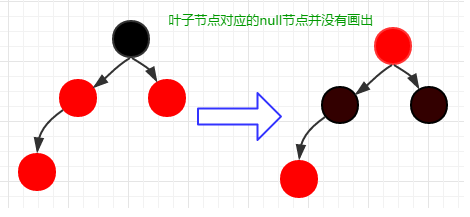调整操作如上图，将父节点和父节点的兄弟节点，都修改为红色，然后将祖父节点修改为红色，因为修改了祖父节点的颜色，祖父节点可能会发生颜色的冲突，所以将新插入的节点修改为祖父节点，在进行调整。

• 情形4：父节点（左孩子节点）的颜色为红色，父节点的兄弟节点的颜色为黑色或者为null，新插入的节点为父节点的右孩子节点。如下图：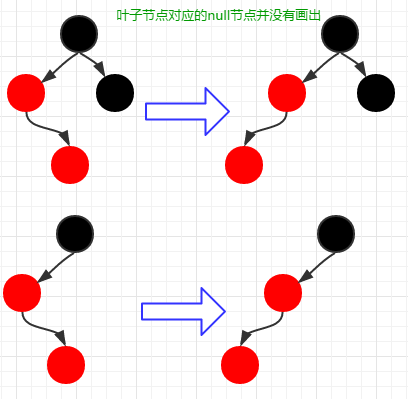此时以父节点为旋转点，就新插入的节点进行左旋操作。便变成了情形5对应的情况，将执行情形5的操作

• 情形5：父节点（左孩子节点）的颜色为红色，父节点的兄弟节点颜色为黑色或者null,新插入节点为父亲的左孩子节点。如下图：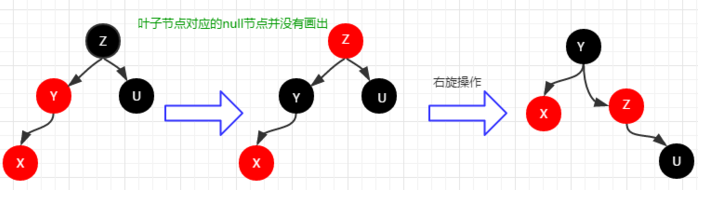• 情形6 和情形7的操作与情形4和情形5的操作相同，它们之前的区别是父节点为有孩子节点，再次不再赘述。

remove()

public V remove(Object key) {
Entry<K,V> p = getEntry(key);  //根据key查找节点，并返回该节点
if (p == null)
return null;

V oldValue = p.value;    //获取key对应的值
deleteEntry(p);     //删除节点
return oldValue;   //返回key对应的值
}

final Entry<K,V> getEntry(Object key) {
//根据键寻找节点，有非为两种方式，如果定制了比较器，采用定制排序方式，否则使用自然排序
if (comparator != null)
return getEntryUsingComparator(key); //循环遍历树，寻找和key相等的节点
if (key == null)
throw new NullPointerException();
Comparable<? super K> k = (Comparable<? super K>) key;
Entry<K,V> p = root;
while (p != null) {  //循环遍历树，寻找和key相等的节点
int cmp = k.compareTo(p.key);
if (cmp < 0)
p = p.left;
else if (cmp > 0)
p = p.right;
else
return p;
}
return null;
}
//删除节点
private void deleteEntry(Entry<K,V> p) {
modCount++;  //记录修改的次数
size--;   //数量减1

//当前节点的两个孩子都不为空
if (p.left != null && p.right != null) {
//寻找继承者，继承者为当前节点的右孩子节点或者右孩子节点的最小左孩子
Entry<K,V> s = successor(p);
p.key = s.key;     //key - value  的替换 ，并没有替换颜色
p.value = s.value;
p = s;  //指向继承者
} // p has 2 children

// Start fixup at replacement node, if it exists.
//开始修复树结构，继承者的左孩子不为空，返回左孩子，否则返回右孩子
//不可能存在左右两个孩子都存在的情况，successor寻找的就是最小节点，它的左孩子节点为null
Entry<K,V> replacement = (p.left != null ? p.left : p.right);

if (replacement != null) {
// Link replacement to parent
//已经被选为继承者，当前拥有的一切放弃，所以将孩子交给爷爷抚养
replacement.parent = p.parent;
//p节点没有父节点，则指向根节点
if (p.parent == null)
root = replacement;
//如果p为左孩子，如果p为左孩子，则将p.parent.left = p.left
else if (p == p.parent.left)
p.parent.left  = replacement;
else
p.parent.right = replacement;

//删除p节点到左右分支，和父节点的引用
p.left = p.right = p.parent = null;

// Fix replacement
if (p.color == BLACK)
//恢复颜色分配
fixAfterDeletion(replacement);
} else if (p.parent == null) { // return if we are the only node.
//红黑书中父节点为空的只能是根节点。
root = null;
} else { //  No children. Use self as phantom replacement and unlink.
if (p.color == BLACK)
fixAfterDeletion(p);

if (p.parent != null) {
if (p == p.parent.left)
p.parent.left = null;
else if (p == p.parent.right)
p.parent.right = null;
p.parent = null;
}
}
}
private void fixAfterDeletion(Entry<K,V> x) {
//不是根节点，颜色为黑色，调整结构
while (x != root && colorOf(x) == BLACK) {

//判断x是否为左孩子
if (x == leftOf(parentOf(x))) {
//x的兄弟节点
Entry<K,V> sib = rightOf(parentOf(x));
//若兄弟节点是红色
if (colorOf(sib) == RED) {
setColor(sib, BLACK);   //设置兄弟节点变为黑色
setColor(parentOf(x), RED);  //父节点设置为红色
rotateLeft(parentOf(x));   //左旋父节点
sib = rightOf(parentOf(x)); //重新设置x的兄弟节点
}

if (colorOf(leftOf(sib))  == BLACK &&
colorOf(rightOf(sib)) == BLACK) {
setColor(sib, RED); //兄弟节点的两个孩子都是黑色的重新设置兄弟节点的颜色，修改为红色
x = parentOf(x);   //将x定位到父节点
} else {
if (colorOf(rightOf(sib)) == BLACK) {   //兄弟节点的右孩子是黑色的，左孩子是红色的
setColor(leftOf(sib), BLACK);  //设置左孩子节点为黑色
setColor(sib, RED); //兄弟节点为红色
rotateRight(sib);   //右旋
sib = rightOf(parentOf(x));  //右旋后重新设置兄弟节点
}
setColor(sib, colorOf(parentOf(x)));  //兄弟节点颜色设置和父节点的颜色相同
setColor(parentOf(x), BLACK);   //父节点设置为黑色
setColor(rightOf(sib), BLACK);  //将兄弟节点的有孩子设置为黑色
rotateLeft(parentOf(x));   //左旋
x = root;  //设置x为根节点
}
} else { // symmetric
//x为父节点的右节点，参考上面的操作
Entry<K,V> sib = leftOf(parentOf(x));

if (colorOf(sib) == RED) {
setColor(sib, BLACK);
setColor(parentOf(x), RED);
rotateRight(parentOf(x));
sib = leftOf(parentOf(x));
}

if (colorOf(rightOf(sib)) == BLACK &&colorOf(leftOf(sib)) == BLACK) {
setColor(sib, RED);
x = parentOf(x);
} else {
if (colorOf(leftOf(sib)) == BLACK) {
setColor(rightOf(sib), BLACK);
setColor(sib, RED);
rotateLeft(sib);
sib = leftOf(parentOf(x));
}
setColor(sib, colorOf(parentOf(x)));
setColor(parentOf(x), BLACK);
setColor(leftOf(sib), BLACK);
rotateRight(parentOf(x));
x = root;
}
}
}

setColor(x, BLACK);
}

• 以排序二叉树的方法删除指定节点。删除的节点存在三种情况：
• 被删除节点，没有左右孩子节点，直接删除即可
• 被删除节点，有一个孩子节点，那么让它的孩子节点指向它的父节点即可
• 本删除的节点，有两个非空的孩子节点，那么需要找到该节点的前驱或者后继节点，更换元素值，在将前驱或者后继节点删除（任意一个节点的前驱或者后继都必定至多有一个非空的子节点，可以按照前面的两种情形进行操作）
• 进行颜色的调换和树的旋转，满足红黑树的特征

• 情形1：被删除的节点为根节点或者颜色为空色，此时删除该节点不影响红黑树的特点。无需操作
• 情形2：被删除节点为黑色，兄弟节点为红色，如下图：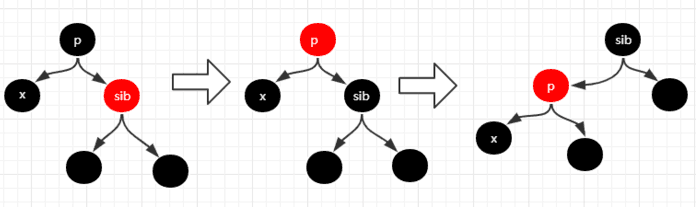若删除上图中的x节点，将缺少一个黑节点，与红黑树的性质冲突，所以修改sib颜色为黑色，设置p节点为红色，并进行左旋操作。在进行后续的处理。

• 情形3：被删除节点为黑色，x节点的兄弟节点的子节点都是黑色，如下图：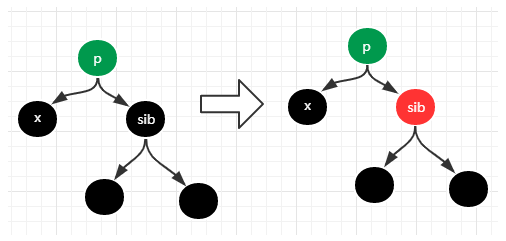x节点是黑色的，兄弟节点（黑色的）的子节点也是黑色的，p节点的颜色无法确定，有可能是红色的，也有可能是黑色的。如果是红色的直接设置为黑色即可，如果为黑色的，则需要将x定位的p节点，在进行处理。

• 情形4：被删除节点为黑色，x的兄弟节点的右自子节点为黑色。如下图：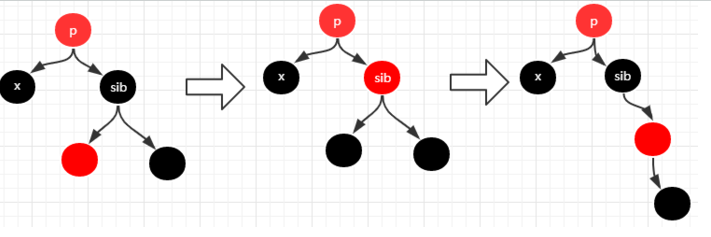情形4的调整为了转变成情形5的情况，来进行处理。

• 情形5：被删除节点为黑色，x的兄弟节点右子节点为红色。如下图：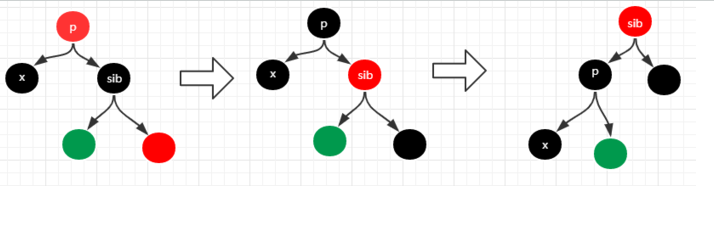sib的左子节点的颜色不确定，可能是红色也可能是黑色，但是对它并没有什么影响，因为变换前后它的上层分支的黑色节点数并没有改变。

• firstEntry() 返回Map中最小的key
• higherEntry(Object key ) 返回该Map中位于key后一位的key-value
• lowerEntry(Object key ) 返回该Map中唯一key前一位的key-value
• tailMap(Object key , boolean inclusive) 返回该Map的子Map

总结

• 关于红黑树的节点插入操作，首先是改变新节点，新节点的父节点，祖父节点，和新节点的颜色，能在当前分支通过节点的旋转改变的，则通过此种操作，来满足红黑书的特点。
• 如果当前相关节点的旋转解决不了红黑树的冲突，则通过将红色的节点移动到根节点解决，最后在将根节点设置为黑色评论(2)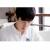if (cpr != null) { //如果定义了比较器，采用自定义比较器进行比较
do {
parent = t; //将红黑树根节点赋值给parent
cmp = cpr.compare(key, t.key); //比较key, 与根节点的大小
if (cmp < 0) //如果key < t.key , 指向左子树
t = t.left; //t = t.left , t == 它的做孩子节点
else if (cmp > 0)
t = t.right; //如果key > t.key , 指向它的右孩子节点
else
return t.setValue(value); //如果它们相等，替换key的值
} while (t != null); //循环遍历
}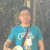(Comparable<? super K>) key; 这样 把 key 类型转换 为 Comparable，如果 k 本身并没有实现 Comparable 接口，这样的 类型转换 是不是会失败啊？

zhoulujun
05/17
58
0
Java核心（四）你不知道的数据集合

2018/11/28
108
0
java集合入门和深入学习，看这篇就差不多了

sihailoveyan
2018/05/04
184
2

mengdonghui123456
2017/08/14
0
0

08/23
0
0

INEVITABLE
17分钟前
4
0
MySQL的COUNT语句，竟然都能被面试官虐的这么惨？

19分钟前
4
0
PHP+Mysql统计文件下载次数实例

PHP+Mysql统计文件下载次数实例，实现的原理也很简单，是通过前台点击链接download.php传参id，来更新点击次数。 获取文件列表： <?php require 'conn.php'; \$query = mysql_query("S...

ymkjs1990
20分钟前
3
0

21分钟前
4
0

linuxCool
21分钟前
4
0